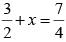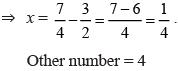Courses

# Olympiad Test: Rational Numbers -1

## 20 Questions MCQ Test Mathematics Olympiad Class 7 | Olympiad Test: Rational Numbers -1

Description
This mock test of Olympiad Test: Rational Numbers -1 for Class 7 helps you for every Class 7 entrance exam. This contains 20 Multiple Choice Questions for Class 7 Olympiad Test: Rational Numbers -1 (mcq) to study with solutions a complete question bank. The solved questions answers in this Olympiad Test: Rational Numbers -1 quiz give you a good mix of easy questions and tough questions. Class 7 students definitely take this Olympiad Test: Rational Numbers -1 exercise for a better result in the exam. You can find other Olympiad Test: Rational Numbers -1 extra questions, long questions & short questions for Class 7 on EduRev as well by searching above.
QUESTION: 1

### By what number should –33/8 be divided to get 11/2?

Solution: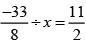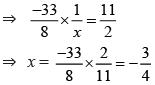QUESTION: 2

### The product of two rational numbers is –10. If one of the numbers is 8, what is the other?

Solution:

8 × (x) = –10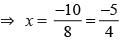QUESTION: 3

### The sum of two rational numbers is –7. If one of the numbers is – 15/6, what is the other?

Solution: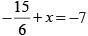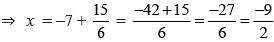QUESTION: 4

Find the additive inverse of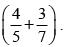Solution: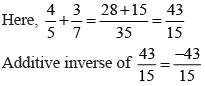QUESTION: 5

What should be added to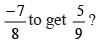Solution: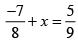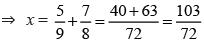QUESTION: 6

What is the value of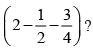Solution: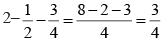QUESTION: 7

What should be added to 2/9 to get –3?

Solution: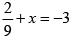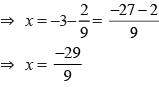QUESTION: 8

The sum of two numbers is 12. If one of them is – 7/9, what is the other?

Solution: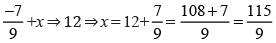QUESTION: 9

What is the simplest form of 84/288?

Solution: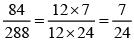QUESTION: 10

Which of the following is correct?

Solution:
QUESTION: 11

What is the value of x if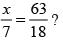Solution: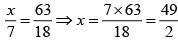QUESTION: 12

By what rational number 2/7 is divided to get 5?

Solution: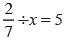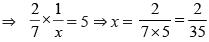QUESTION: 13

By what rational number 3/13 is multiplied to get –12?

Solution: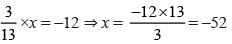QUESTION: 14

Which of the following is incorrect?

Solution: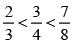QUESTION: 15

Which rational number is in between 2 and 3?

Solution:
QUESTION: 16

The cost of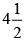metres of cloth is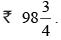What is the cost of cloth per metre?

Solution:

Cost of cloth per metre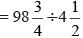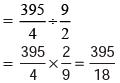QUESTION: 17

What is the simplified value of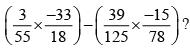Solution: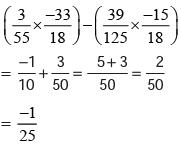QUESTION: 18

What is the reciprocal of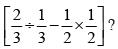Solution: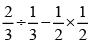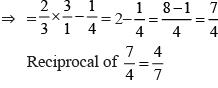QUESTION: 19

The reciprocal of a rational number is –7/9. What is that rational number?

Solution: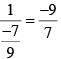QUESTION: 20

The sum of reciprocals of two rational numbers is 7/4. If one of the numbers is 2/3 what is the other?

Solution: